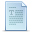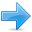# Constants

Constants are expressions with a fixed value.

### Literals

Literals are the most obvious kind of constants. They are used to express particular values within the source code of a program. We have already used these previously to give concrete values to variables or to express messages we wanted our programs to print out, for example, when we wrote:

 ``1`` ``a = 5;``

the `5` in this piece of code was a literal constant.

Literal constants can be divided in Integer Numerals, Floating-Point Numerals, Characters, Strings and Boolean Values.

#### Integer Numerals

 ``123`` ``````1776 707 -``````

They are numerical constants that identify integer decimal values. Notice that to express a numerical constant we do not have to write quotes (`"`) nor any special character. There is no doubt that it is a constant: whenever we write `1776` in a program, we will be referring to the value 1776.

In addition to decimal numbers (those that all of us are used to using every day), C++ allows the use of octal numbers (base 8) and hexadecimal numbers (base 16) as literal constants. If we want to express an octal number we have to precede it with a `0` (a zero character). And in order to express a hexadecimal number we have to precede it with the characters `0x` (zero, x). For example, the following literal constants are all equivalent to each other:

 ``123`` ``````75 // decimal 0113 // octal 0x4b // hexadecimal ``````

All of these represent the same number: 75 (seventy-five) expressed as a base-10 numeral, octal numeral and hexadecimal numeral, respectively.

Literal constants, like variables, are considered to have a specific data type. By default, integer literals are of type int. However, we can force them to either be unsigned by appending the u character to it, or long by appending l:

 ``1234`` ``````75 // int 75u // unsigned int 75l // long 75ul // unsigned long ``````

In both cases, the suffix can be specified using either upper or lowercase letters.

#### Floating Point Numbers

They express numbers with decimals and/or exponents. They can include either a decimal point, an `e` character (that expresses "by ten at the Xth height", where X is an integer value that follows the `e` character), or both a decimal point and an `e` character:

 ``1234`` ``````3.14159 // 3.14159 6.02e23 // 6.02 x 10^23 1.6e-19 // 1.6 x 10^-19 3.0 // 3.0 ``````

These are four valid numbers with decimals expressed in C++. The first number is PI, the second one is the number of Avogadro, the third is the electric charge of an electron (an extremely small number) -all of them approximated- and the last one is the number three expressed as a floating-point numeric literal.

The default type for floating point literals is double. If you explicitly want to express a float or a long double numerical literal, you can use the `f` or `l` suffixes respectively:

 ``12`` ``````3.14159L // long double 6.02e23f // float ``````

Any of the letters that can be part of a floating-point numerical constant (`e`, `f`, `l`) can be written using either lower or uppercase letters without any difference in their meanings.

#### Character and string literals

There also exist non-numerical constants, like:

 ``1234`` ``````'z' 'p' "Hello world" "How do you do?"``````

The first two expressions represent single character constants, and the following two represent string literals composed of several characters. Notice that to represent a single character we enclose it between single quotes (`'`) and to express a string (which generally consists of more than one character) we enclose it between double quotes (`"`).

When writing both single character and string literals, it is necessary to put the quotation marks surrounding them to distinguish them from possible variable identifiers or reserved keywords. Notice the difference between these two expressions:

 ``12`` ``````x 'x'``````

`x` alone would refer to a variable whose identifier is `x`, whereas `'x'` (enclosed within single quotation marks) would refer to the character constant 'x'.

Character and string literals have certain peculiarities, like the escape codes. These are special characters that are difficult or impossible to express otherwise in the source code of a program, like newline (`\n`) or tab (`\t`). All of them are preceded by a backslash (`\`). Here you have a list of some of such escape codes:

 `\n` newline `\r` carriage return `\t` tab `\v` vertical tab `\b` backspace `\f` form feed (page feed) `\a` alert (beep) `\'` single quote (') `\"` double quote (") `\?` question mark (?) `\\` backslash (\)

For example:

 ``1234`` ``````'\n' '\t' "Left \t Right" "one\ntwo\nthree"``````

Additionally, you can express any character by its numerical ASCII code by writing a backslash character (`\`) followed by the ASCII code expressed as an octal (base-8) or hexadecimal (base-16) number. In the first case (octal) the digits must immediately follow the backslash (for example `\23` or `\40`), in the second case (hexadecimal), an `x` character must be written before the digits themselves (for example `\x20` or `\x4A`).

String literals can extend to more than a single line of code by putting a backslash sign (`\`) at the end of each unfinished line.

 ``12`` ``````"string expressed in \ two lines"``````

You can also concatenate several string constants separating them by one or several blank spaces, tabulators, newline or any other valid blank character:

 ``1`` ``"this forms" "a single" "string" "of characters"``

Finally, if we want the string literal to be explicitly made of wide characters (wchar_t type), instead of narrow characters (char type), we can precede the constant with the `L` prefix:

 ``1`` ``L"This is a wide character string"``

Wide characters are used mainly to represent non-English or exotic character sets.

#### Boolean literals

There are only two valid Boolean values: true and false. These can be expressed in C++ as values of type bool by using the Boolean literals `true` and `false`.

### Defined constants (#define)

You can define your own names for constants that you use very often without having to resort to memory-consuming variables, simply by using the `#define` preprocessor directive. Its format is:

`#define identifier value`
For example:

 ``12`` ``````#define PI 3.14159 #define NEWLINE '\n' ``````

This defines two new constants: PI and NEWLINE. Once they are defined, you can use them in the rest of the code as if they were any other regular constant, for example:

 ``12345678910111213141516171819202122232425262728293031`` ``````// defined constants: calculate circumference #include using namespace std; #define PI 3.14159 #define NEWLINE '\n' int main () { double r=5.0; // radius double circle; circle = 2 * PI * r; cout << circle; cout << NEWLINE; return 0; }`````` `31.4159`

In fact the only thing that the compiler preprocessor does when it encounters `#define` directives is to literally replace any occurrence of their identifier (in the previous example, these were PI and NEWLINE) by the code to which they have been defined (`3.14159` and `'\n'` respectively).

The `#define` directive is not a C++ statement but a directive for the preprocessor; therefore it assumes the entire line as the directive and does not require a semicolon (`;`) at its end. If you append a semicolon character (`;`) at the end, it will also be appended in all occurrences of the identifier within the body of the program that the preprocessor replaces.

### Declared constants (const)

With the `const` prefix you can declare constants with a specific type in the same way as you would do with a variable:

 ``12`` ``````const int pathwidth = 100; const char tabulator = '\t';``````

Here, `pathwidth` and `tabulator` are two typed constants. They are treated just like regular variables except that their values cannot be modified after their definition.Previous:Variables. Data Types.IndexNext:Operators